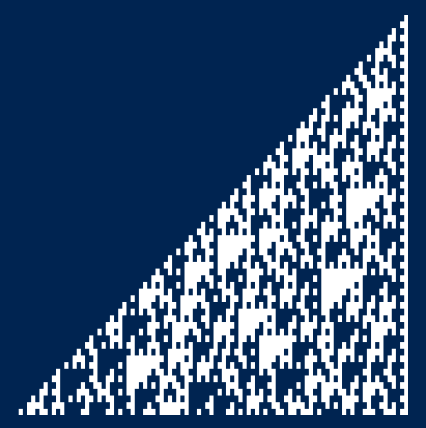# Powers of 3 in binary

I was thumbing through A New Kind of Science  and one of the examples that jumped out at me was looking at the bits in the binary representation of the powers of 3. I wanted to reproduce the image myself and here’s the result.Here a white square represents a 1 and a blue square a 0. There’s a white border on the right edge because all powers of 3 are odd, i.e. end in 1 when written in binary.

As with many of the images in Wolfram’s book, it’s somewhere between regular and chaotic.

I produced the image at the command line with the following Python code.

```    from numpy import log2

N = 60
k = int(log2(3)*N)+1

for i in range(N):
s = format(3**i, str(k)+'b')
s = s.replace('1', chr(0x2588))
s = s.replace('0', ' ')
print(s)
```

The code writes powers of three as binary strings of length k where k is calculated so the last number in the sequence will fit. It then replaces 1s with a solid block character and replaces 0s with a blank space. My terminal has a blue background and a white foreground, so 1s show up as white squares and 0s as blue.

The characters in my terminal are twice as high as they are wide, so I changed the aspect ratio after taking the screen shot so that the blocks come out more square.

## Update: Other bases

What if we look at bases other than 3?

Here’s the revised code to let you specify any base b.

```    from numpy import log2

def out(s):
s = s.replace('1', chr(0x2588))
s = s.replace('0', ' ')
print(s)

b = 5
N = 50
k = int(log2(b)*N)+1

for i in range(N):
s = format(b**i, str(k)+'b')
out(s)
```

Here’s what powers of 5 look like:And here’s what powers of 7 look like:What if our base isn’t prime? It doesn’t make an obvious difference if the base is odd, but if it’s even we get a skewed image. Here’s the plot for p = 6.This is just the plot for powers of 3 with the nth row shifted left by n positions.

## Related posts

 I’ve looked through the book before for fun, but this time I’m looking through it with a practical project in mind. I never thought that would happen.

## 6 thoughts on “Powers of 3 in binary”

1. You pushed me over the edge to finally get my own copy of NKoS.

Not yet sure if I’ll thank you for that or not…

2. The MOD operator makes all kinds of interesting patterns when applied to tables of numbers. For example, you can take Pascal’s triangle “MOD 2” to obtain a (rotated) version of Sierpinski’s triangle. Or apply MOD 3 to Pascal’s triangle to obtain a three-number pattern. Some pictures at https://blogs.sas.com/content/iml/2014/12/03/pascals-triangle-in-sas.html

3. Nice paper. Thanks.

4. Nathan Hannon

“This is just the plot for powers of 2 with the nth row shifted left by n positions.”

I think that should be “powers of 3”. (Powers of 2 in binary, it turns out, don’t look very chaotic.)

5. @Nathan, no, not very chaotic at all. :)

Thanks.